# Finding the side length of a rectangle given its perimeter or area Online Quiz

#### Complete Python Prime Pack

9 Courses     2 eBooks

#### Artificial Intelligence & Machine Learning Prime Pack

6 Courses     1 eBooks

#### Java Prime Pack

9 Courses     2 eBooks

Following quiz provides Multiple Choice Questions (MCQs) related to Finding the side length of a rectangle given its perimeter or area. You will have to read all the given answers and click over the correct answer. If you are not sure about the answer then you can check the answer using Show Answer button. You can use Next Quiz button to check new set of questions in the quiz.Q 1 - Determine the value of 'L'.

Area = 24 square cm### Explanation

Step 1:

Area of a rectangle = l × w; l = length = L; w = width = 6

Step 2:

Area of given rectangle = L × 6 = 24 square cm

Missing length L = $\frac{24}{6}$ = 4 cm

Q 2 - Determine the value of 'L'.

Area = 30 square cm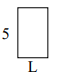### Explanation

Step 1:

Area of a rectangle = l × w; l = length = L; w = width = 5

Step 2:

Area of given rectangle = L × 5 = 30 square cm

Missing length L = $\frac{30}{5}$ = 6 cm

Q 3 - Determine the value of 'L'.

Perimeter = 22 cm### Explanation

Step 1:

Perimeter of a rectangle = 2(l + w); l = length = L; w = width = 6

Step 2:

Perimeter of given rectangle = 2(L + 6) = 22 cm

L + 6 = $\frac{22}{2}$ = 11;

So missing length L = 11 – 6 = 5 cm

Q 4 - Determine the value of 'L'.

Perimeter = 20 cm### Explanation

Step 1:

Perimeter of a rectangle = 2(l + w); l = length = L; w = width = 8

Step 2:

Perimeter of given rectangle = 2(L + 8) = 20 cm

L + 8 = $\frac{20}{2}$ = 10;

So missing length L = 10 – 8 = 2 cm

Q 5 - Determine the value of 'L'.

Area = 25 square cm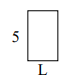### Explanation

Step 1:

Area of a rectangle = l × w; l = length = L; w = width = 5

Step 2:

Area of given rectangle = L × 5 = 25 square cm

Missing length L = $\frac{25}{5}$ = 5 cm

Q 6 - Determine the value of 'L'.

Perimeter = 20 cm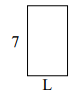### Explanation

Step 1:

Perimeter of a rectangle = 2(l + w); l = length = L; w = width = 7

Step 2:

Perimeter of given rectangle = 2(L + 7) = 20 cm

L + 7 = $\frac{20}{2}$ = 10;

So missing length L = 10 – 7 = 3 cm

Q 7 - Determine the value of 'L'.

Area = 30 square cm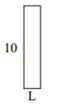### Explanation

Step 1:

Area of a rectangle = l × w; l = length = L; w = width = 10

Step 2:

Area of given rectangle = L × 10 = 30 square cm.

Missing length L = $\frac{30}{10}$ = 3 cm

Q 8 - Determine the value of 'L'.

Perimeter = 16 cm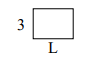### Explanation

Step 1:

Perimeter of a rectangle = 2(l + w); l = length = L; w = width = 3

Step 2:

Perimeter of given rectangle = 2(L + 3) = 16 cm

L + 3 = $\frac{16}{2}$ = 8;

So missing length L = 8 – 3 = 5 cm

Q 9 - Determine the value of 'L'.

Perimeter = 30 cm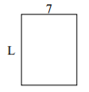### Explanation

Step 1:

Perimeter of a rectangle = 2(l + w); l = length = L; w = width = 7

Step 2:

Perimeter of given rectangle = 2(L + 7) = 30 cm

L + 7 = $\frac{30}{2}$ = 15;

So missing length L = 15 – 7 = 8 cm

Q 10 - Determine the value of 'L'.

Area = 40 square cm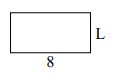### Explanation

Step 1:

Area of a rectangle = l × w; l = length = L; w = width = 8

Step 2:

Area of given rectangle = L × 8 = 40 square cm

Missing length L = $\frac{40}{8}$ = 5 cm

finding_side_length_of_rectangle_given_its_perimeter_or_area.htm